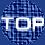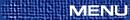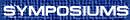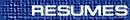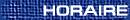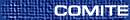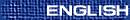Contributed Papers Session / Communications libres(Patrick Browne, Organizer) KHALID EL-YASSINI Some interior-exterior points methods for linear programming We describe different interior-exterior algorithms for linear programming problem and we specially present our recent algorithm based on path following idea. The algorithm uses a two parameter mixed penalty function. Each iteration updates the penalty parameters. An approximate solution, of Karush-Kuhn-Tucker system of equations which characterizes a solution of the mixed penalty function, is computed by using only one Newton direction or by using the predictor-corrector method. The approximate solution obtained gives a dual and a pseudo-feasible primal points. Since the primal solution is non feasible, a new pseudo-gap definition is introduced to characterize primal and dual solutions. Finally, Some numerical results will be presented. ILHAN IZMIRLI, Strayer University, Washington, D.C.  20005, USA On some generalizations of an indeterminate problem of Ibn Hamza In this paper, I will talk about some of the contributions of the famous Middle Eastern mathematician Ibn Hamza to mathematics. I will mostly concentrate on a problem posed and solved by him around 1590's, along with some interesting generalizations of this rather elegant solution. JOHN H. URSELL, P.O. Box 761, Kingston, Ontario  K7L 4X6 An elementary proof of the second case of Fermat's last theorem Fermat's Last Theorem is divided into exactly two cases for odd prime exponent p. This talk gives an elementary proof of the second case. The present author believes that Pierre de Fermat had this proof. YOUCHEN ZHOU, Zhejian University On Moeckel-like boundary of the local Siegel disk For a family of simple closed curves in the local Siegel Disk (defined by J. T. Rogers, Jr.) that converges to the boundary of the disk, we get a simple and clear condition on it that is sufficient and necessary to the boundary being Moeckel-like. It is also proven that third and fourth kinds of prime ands are empty for Moeckel-like boundary.